Hello Guest it is October 24, 2021, 03:50:22 AM

###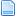Author Topic: Using Formula Axis Correction to engrave on a cylinder  (Read 8603 times)

0 Members and 1 Guest are viewing this topic.

####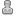ChrisLT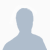•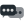19##### Using Formula Axis Correction to engrave on a cylinder
« on: September 04, 2007, 10:30:46 AM »
I'm using Formulas to wrap 2D engravings (e.g. HPGL and DXF imports) and text (e.g. the Write wizard) around curved surfaces such as cylinders, using a 3 axis machine.

Formula Axis Correction seems to have absolutely no documentation, so I'll start by stating my understanding of how it works.
With Formulas enabled, all gcode moves have the formulae applied before execution. The (X,Y,Z,A,B,C) endpoint calculated by the formulae is then used as the destination of the move. Mach3 does linear interpolation from the current point to this endpoint. The axis DROs show the physical mapped coordinates, not the coordinates commanded by the gcode. Jog buttons continue to move only one axis.
I find this display by the DROs potentially confusing. It would be nice also to be able to see the logical coordinates. For instance, in MDI mode, if the Z DRO shows -1 and you command G0 Z0 you might be surprised if the tool moves downwards.

Anyway, my first use of this feature is to engrave on the surface of a cylinder, using 2D engraving gcode, such as the Write wizard or DXF or HPGL import. By telling Mach3 that the Z height is affected by the X or Y value, I can make it curve the engraving correctly so it is at constant depth.
Start by fixing the workpiece parallel to the X (or Y) axis. Set Y (or X) and Z to zero with the tool centred above and just brushing the top of the work.
In Config>Formulas... set:
f(y)=(R+z)*sin(y/R)
f(z)=(R+z)*cos(y/R)-R

...where R is the radius of the cylindrical workpiece. This makes y equal to the distance around the circumference. For workpieces that are only curved a little, you'll have to calculate the radius from the curvature.

With a 3 axis machine, the tool is going to remain vertical, so you don't want to use this method too far off axis except with a ball-ended tool.

As Mach3 does linear interpolation, it will gouge if you do a long cutting move, but for engraving text this hasn't been a problem for me. To draw a box around the text, you have to split the circumferential lines into little pieces. And don't be tempted to jog.

The same method can be used to engrave on the inside of a curved surface. Use:
f(y)=(R-z)*sin(y/R)
f(z)=R-(R-z)*cos(y/R)

As I'm not using the A, B and C axes on my 3-axis machine, I believe I could use any of them to hold R, making it a variable, as in:
f(y)=(a-z)*sin(y/a)

...where A can be set by G0 A1000 for instance, and can be seen in a DRO, which is helpful.

If the radius is great and the curvature is slight, you can simplify the equations. Use:
f(y)=y
f(z)=z-y*y/(2*R)

I'm very new here, so perhaps these ideas are already known, and maybe there are issues I haven't yet run into, but I couldn't find them written up anywhere.

Chris Lusby Taylor

####Chaoticone•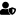•5,626
• Precision Chaos##### Re: Using Formula Axis Correction to engrave on a cylinder
« Reply #1 on: September 04, 2007, 01:19:09 PM »
Thanks for shareing that Chris.

BrettIf you could see the things I have in my head, you would be laughing too.My guard dog is not what you need to worry about!